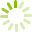# Calculus 2 (MATH 1200)

This course is designed to provide students with a fundamental knowledge of integral calculus. Topics include antidifferentiation; the definite integral; the fundamental theorem of calculus, areas and volumes; integration techniques; improper integrals; applications of the integral; slope fields; numerical approximations; linear differential equations and applications; polynomial approximations; Taylor series, power series and calculus with parametric curves and polar coordinates.
Course code: MATH 1200
Credits: 3.0
Length: 60.0 hours
Course outline: view https://www.vcc.ca/vccphp/courseoutline?subject=MATH&number=1200

## Prerequisites

MATH 1100 with a minimum 'C-' grade.